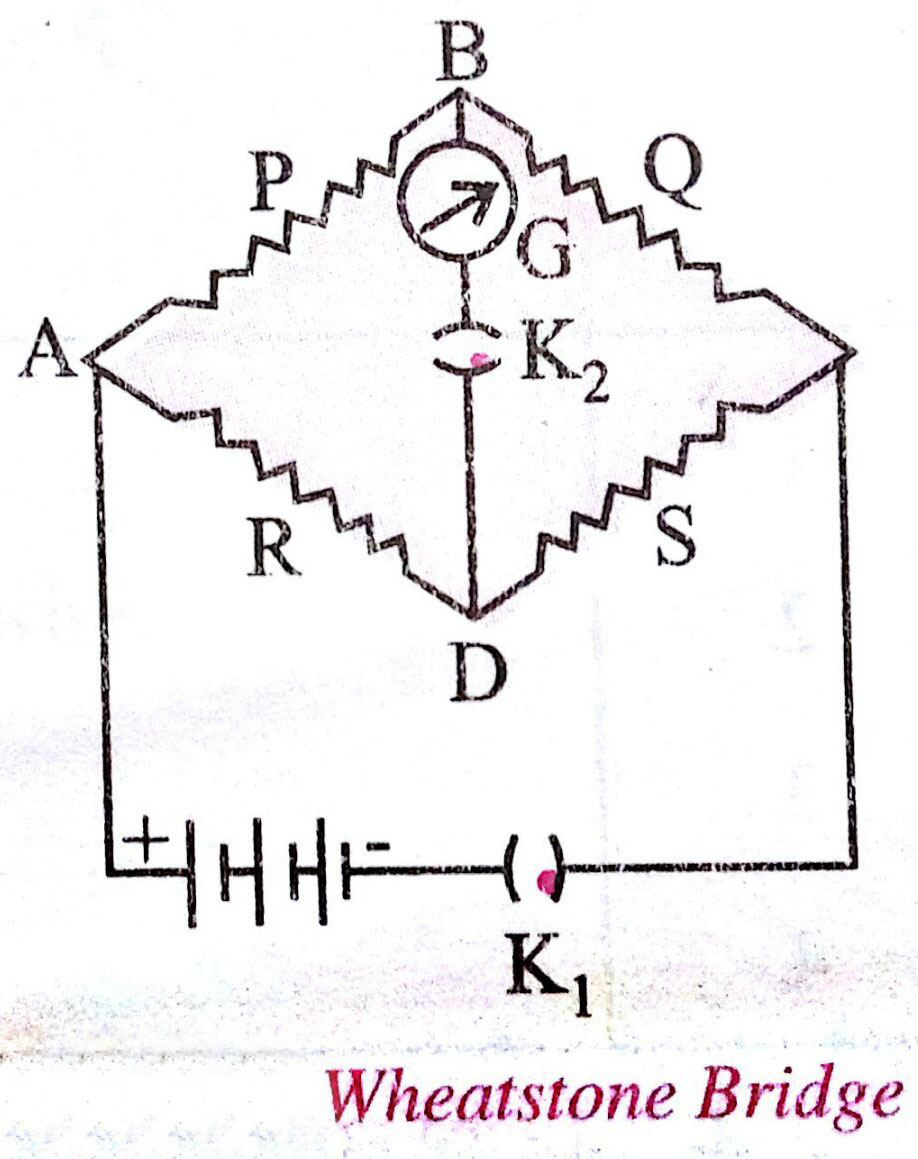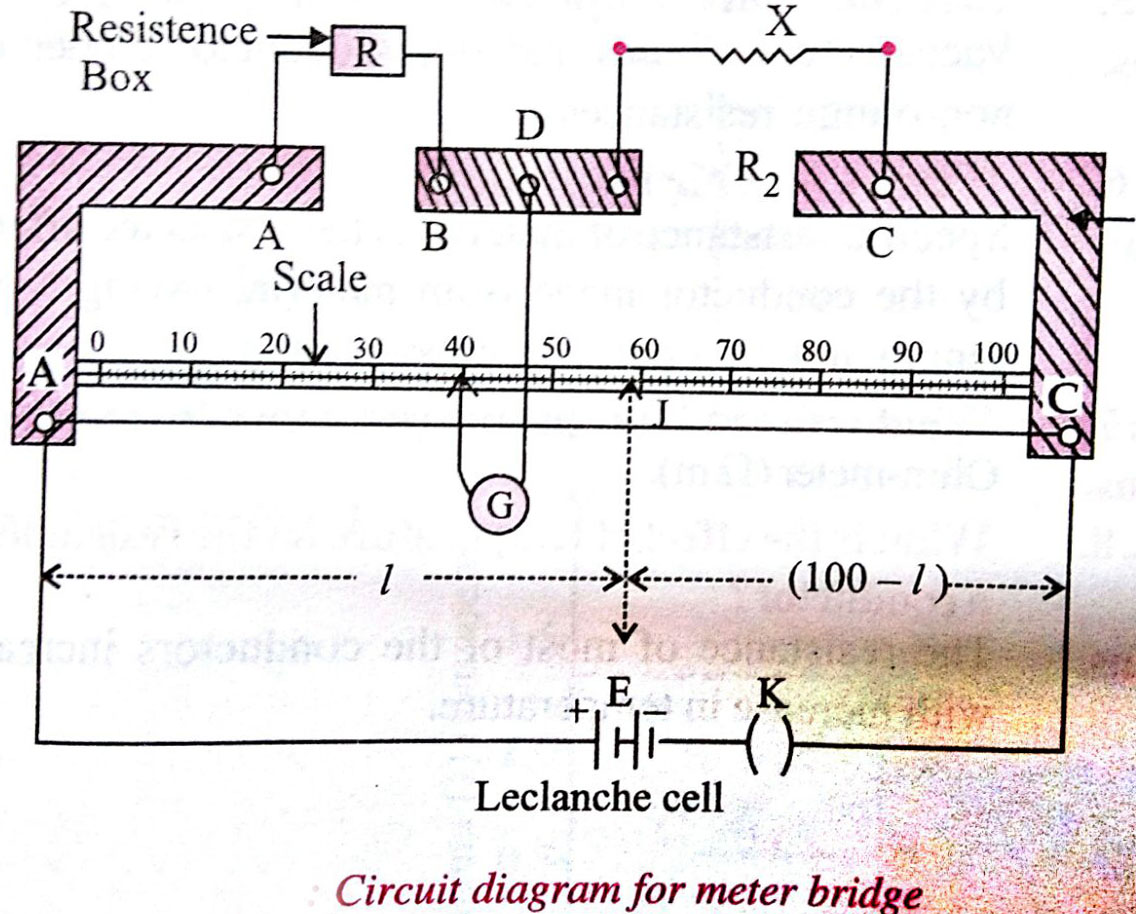### Which of the following statements is false ?

• Option 1)

Wheatstone bridge is the most sensitive when all the four resistances are of the same order of magnitude.

• Option 2)

In a balanced wheatstone bridge if the cell and the galvanometer are exchanged, the null point is disturbed.

• Option 3)

A rheostat can be used as a potential divider.

• Option 4)

Kirchhoff’s second law represents energy conservation.As we learnt in

To find resistance of a given wire using metre bridge and hence determine the specific resistance -For unknown resistancex= Unknown resistance

R= Given resistance

- wherein

Calculation= .........ohms= Resistivity

X = Unknown resistance

D = Diametre

L = length of wire

There is no change in null point, if the cell and the galvanometer are exchanged in a balanced bridge.

So, option (2) is the answer

Balancing conditionBalancing conditionOption 1)

Wheatstone bridge is the most sensitive when all the four resistances are of the same order of magnitude.

Incorrect

Option 2)

In a balanced wheatstone bridge if the cell and the galvanometer are exchanged, the null point is disturbed.

Correct

Option 3)

A rheostat can be used as a potential divider.

Incorrect

Option 4)

Kirchhoff’s second law represents energy conservation.

Incorrect

#### divya.saini